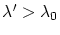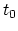Next: The Mach principle Up: The basis of the GRT Previous: Some GRT corollaries   Contents

# Criticism of the relativistic cosmology

The theories of evolution of the Universe will remain the hypotheses for ever, because none of assumptions (even on the isotropy and homogeneity) can be verified: "a moving train, which departed long ago, can be catched up only at the other place and at the other time". GRT assigns to itself the resolution of a series of paradoxes (gravitational, photometric, etc.). Recall that the gravitational paradox consists in the following: it is impossible to obtain the definite value of the gravitational acceleration of a body from Poisson's equation for the infinite Universe possessing a uniform density. (What relationship to the reality bore pure mathematical uncertainties with conditions on infinity for a physical model?) Recall also the essence of the photometric paradox: for the infinitely existing (stationary) infinite Universe the brightness of sky must be equal to the mean brightness of stars without considering the light absorption and transform (again we have rather many unreal assumptions). However, the classical physics has also described the possibilities of resolution of similar paradoxes (for example, by means of systems of different orders: Emden's spheres, Charlier's structures, etc.). Apparently, the Universe is not a spread medium, and we do not know at all its structure as a whole to assert the possibility of realization of conditions for similar paradoxes (more probably, the opposite situation is true). For example, the Olbers photometric paradox can easily be understood on the basis of the analogy with the ocean: the light is absorbed, scattered and reflected by portions, and the light simply ceases to penetrate to a particular depth. Certainly, such a "depth" is huge for the rarefied Universe. However, the flashing stars represent rather compact objects spaced at great distances from each other. As a result, only a finite number of stars make a contribution into the light intensity of the night sky (to say nothing of the fact that the Doppler effect, or, more better, the red shift as the experimental fact, must be also taken into account in the theory).

The situation with the red shift in spectra of astronomical objects does not be finally clarified. In the Universe there exists a considerable number of objects with quite different shifts in different spectral regions. Generally speaking, since distances to remote objects do not directly measured (the calculated result is connected with some hypotheses), then their relation with the red shift is hypothesis also (for which it is unknown what the matter could be verified). For example, the expanding of the Universe gives a red shift according to the Doppler effect irrespective of GRT. Besides, it should be taken into consideration, that even the elementary scattering will make contribution into the red shift and filling of the so-called relic radiation: recall that the Compton effect gives waves with. The shift of lines in the gravitational field has been well predicted even by mechanistic models from the general energy considerations.

Generally speaking, the theory of Big Bang casts the Big doubts. In addition to the banal questions: "what, where and when was exploded" (since space, time and substance did not exist), the question arises: what about the GRT conclusion on black holes (and the insuperability of light speed)? At the time origin the Universe must be a black hole (and not only at this time instant, but throughout some period). Since we observe the occurring everywhere expansion, the GRT limitations and rather figurative description of compression for black holes disappeared somewhere. Probably, it is interesting to invent something that cannot be verified (only it does not worth to name the science).

Now we pass to the following principal issue. Whether positive is the fact, that the distribution and motion of the matter cannot be specified arbitrarily? And whether is it correct? Generally, this implies the inconsistency of the theory, because there exist other forces, except gravitational ones, which are also capable to transpose the matter. From the practical viewpoint this means, that we should specify all distributions in the "correct-for-GRT" manner even at the initial time instant. In such a case we should referinstant to "the time of creation", did we? And what principles should be unambiguously determinate for such a choice? This requires more knowledge, than results are expected from GRT. Open to question occurs to be the possibility of point-like description and the theory of disturbances, because the resulting values cannot be arbitrary as well. The joining of a completely unknown equation of state to the system of equations implies artificial complication of macro- and micro-levels by linkage and reflects the possibility of arbitrary fittings (for example, the temperature dependence is rejected). The possibility of adding the cosmological constant into Einstein's equations is an indirect recognition of ambiguity of GRT equations and of possible outrage. If everything can be specified to such an accuracy, then why cannot we specify in arbitrary manner the initial distribution and the motion of a matter?

SubsectionsNext: The Mach principle Up: The basis of the GRT Previous: Some GRT corollaries   Contents
Sergey N. Arteha